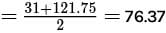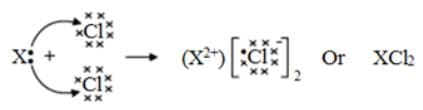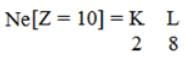# Test: Periodic Classification of Elements (Medium)

## 20 Questions MCQ Test Additional Documents & Tests for Class 10 | Test: Periodic Classification of Elements (Medium)

Description
Attempt Test: Periodic Classification of Elements (Medium) | 20 questions in 20 minutes | Mock test for Class 10 preparation | Free important questions MCQ to study Additional Documents & Tests for Class 10 for Class 10 Exam | Download free PDF with solutions
QUESTION: 1

### An atom has the symbol pqX. Which value determines the position of the element in the periodic table?

Solution: q is the atomic number. It determines the number of electrons, valence electrons and hence the period and the group number.

QUESTION: 2

### Which of the following is not a noble gas?

Solution: Helium, xenon, and radon are inert gases. Radium is an alkaline earth metal.
QUESTION: 3

### The element placed with cerium, in the table formed by Newlands' was:

Solution: The element placed with cerium, in Newlands' table, was lanthanum.

QUESTION: 4

Element 'X' has twelve protons in its nucleus. To which group of the periodic table it will belong?

Solution: The element has 12 protons. Thus its atomic number is 12. The element of atomic number 12 is Mg Magnesium belongs to second group.
QUESTION: 5

Which among the following elements is metalloid?

Solution: Boron is element which has properties in between metals and non-metals. Hence, Boron is metalloid. Option C is correct.
QUESTION: 6

Law of Triad was proposed by

Solution: In 1829, a German chemist, Johann Dobereiner (1780–1849), placed various groups of three elements into groups called triads. One such triad was lithium, sodium, and potassium. Triads were based on both physical as well as chemical properties.
QUESTION: 7

Which of the following statements can help a chemistry student to predict chemical properties of an element?

(I) Position of element in the periodic table

(II) Atomic number of the element

(III) Number of shells in the atom

(IV) Number of electrons in the outermost shell.

Solution: To predict chemical properties of an element.
QUESTION: 8

If the two members of a Dobereiner triad are phosphorus and antimony, the third member of this triad is:

Solution: According to Dobereiner, the atomic mass of the middle element was nearly equal to the arithmetic mean of the other two. Therefore, the atomic mass of the third element iswhich is nearly equal to the atomic mass of arsenic.
QUESTION: 9

_________were not included in Mendeleev's periodic table.

Solution: There were many demerits in the Mendeleev periodic table, such as isotopes were not included in his periodic table as the isotopes were discovered long after this discovery.
QUESTION: 10

Whose name is not associated with the development of periodic Table

Solution: William Prout discovered that the atomic mass of an element can never differ. He observed that many elements have atomic weights as multiple of that of hydrogen. Newland and Loother Meyer also played a great role in the attempts to classify the elements. However, Rutherford played a prominent role in explaining the structure of the atom. Therefore, the correct answer is (c).
QUESTION: 11

Which of the following forms the basis of the modern periodic table?

Solution: Modern periodic table is based on the atomic numbers of elements as according to the modern periodic law the properties of elements are a periodic function of their atomic numbers.
QUESTION: 12

What happens to the electropositive character of elements moving from left to right in a periodic table?

Solution: Electropositive character of an element is its ability to lose electrons and form positive ions. Now, as on moving from left to right in a period of periodic table, the nuclear charge increases due to the gradual increase in number of protons, so the valence electrons are pulled more strongly by the nucleus. Thus, it becomes more and more difficult for the atoms to lose electrons causing a decrease in the electropositive character of elements moving from left to right in a periodic table.
QUESTION: 13

The electronic configuration of an element M is 2, 8, 4. In modern periodic table, the element M is placed in

Solution: In the periodic table, elements having 4 valence electrons are placed in group 14.
QUESTION: 14

Which of the following is the correct order of the atomic radii of the elements oxygen, fluorine and nitrogen?

Solution: Oxygen (8), fluorine (9), and nitrogen (7) belong to the same period of the periodic table, in the order nitrogen, oxygen, and fluorine. Now in a period, on moving from left to right the atomic radius of the elements decreases. Therefore, the atomic radius of nitrogen is the largest.
QUESTION: 15

What is the other name for group 18th elements?

Solution: Group 18th elements are named as noble gases as they are very stable due to having the maximum number of valence electrons their outermost shell can hold, hence they rarely react with other elements.
QUESTION: 16

Which of the following is the most reactive element of the group 17?

Solution: As we move down in a group, the size of the atoms of elements goes on increasing. So, fluorine being on the top position in the halogen’s group is the smallest element and has the maximum tendency to gain an electron to complete its octet. Thus fluorine is the most reactive element of the group 17.
QUESTION: 17

Element X forms a chloride with the formula XCl2, which is a solid with a high melting point. X would most likely be in the same group of the Periodic Table as

Solution: Group 2 alkaline earth metal atoms have two valence electrons each. They can donate their two valence electrons to two other chlorine atoms to form the solid compounds of the form XCl2.This XCl2 compound being ionic in nature has a very strong electrostatic force of attraction between 2 chloride atoms and 1 metal atom. Thus a large amount of heat is required to break these strong bonds, causing the compound to have very high melting and boiling points.

QUESTION: 18

Which group elements are called transition metals?

Solution: The elements occurring in the group 3 to 12 are named as transition metals because they are metallic elements that form a transition between the main group elements, which occur in groups 1 and 2 on the left side, and groups 13–18 on the right side of the periodic table.
QUESTION: 19

Which of the following elements has 2 shells and both are completely filled?

Solution: Neon with the atomic number 10, has the electronic configuration asHence, both its K and L shells are completely filled.

QUESTION: 20

Which of the following is the atomic number of an element that forms basic oxide?

Solution: The elements which can donate their valence electrons to other atoms are the metallic elements which form basic oxides as they give hydroxides in their aqueous solutions.Use Code STAYHOME200 and get INR 200 additional OFF Use Coupon Code